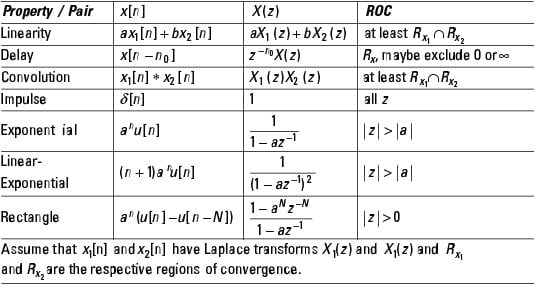##### Signals and Systems For Dummies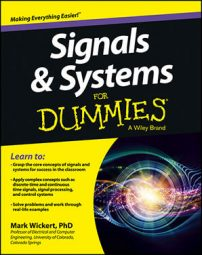Both signals and systems can be analyzed in the time-, frequency-, and s- and z-domains. Leaving the time-domain requires a transform and then an inverse transform to return to the time-domain.

As you work to and from the time domain, referencing tables of both transform theorems and transform pairs can speed your progress and make the work easier. Use this table of common pairs for the continuous-time Fourier transform, discrete-time Fourier transform, the Laplace transform, and the z-transform as needed.

Working in the frequency domain means you are working with Fourier transform and discrete-time Fourier transform — in the s-domain.

## Using Fourier transforms for continuous-time signals

Here is a short table of theorems and pairs for the continuous-time Fourier transform (FT), in both frequency variable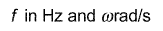The forward and inverse transforms for these two notational schemes are defined as: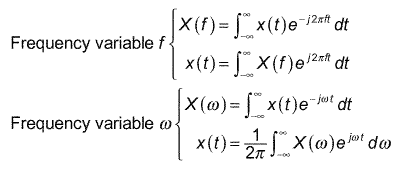. . . and here's the table: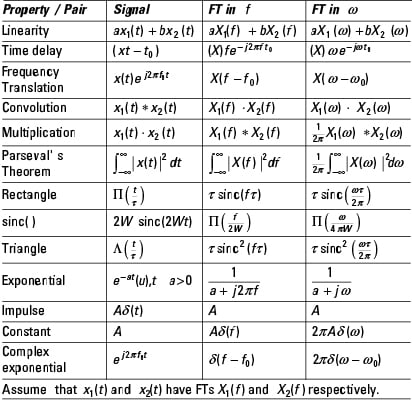## Applying Fourier transform to discrete-time signals

For discrete-time signals and systems the discrete-time Fourier transform (DTFT) takes you to the frequency domain. A short table of theorems and pairs for the DTFT can make your work in this domain much more fun. The discrete-time frequency variable is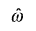The forward and inverse transforms are defined as: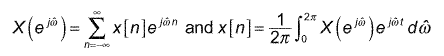. . . and here's the table: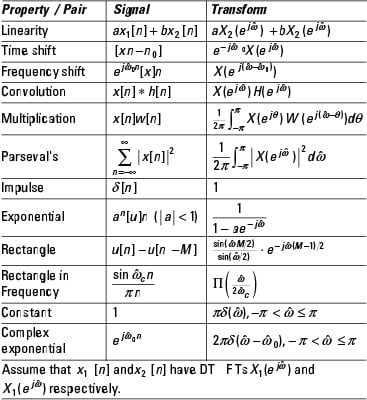## Using the Laplace transform in the s-domain

For continuous-time signals and systems, the one-sided Laplace transform (LT) helps to decipher signal and system behavior. It's also the best approach for solving linear constant coefficient differential equations with nonzero initial conditions. The one-sided LT is defined as: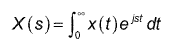The inverse LT is typically found using partial fraction expansion along with LT theorems and pairs. Here's a short table of LT theorems and pairs.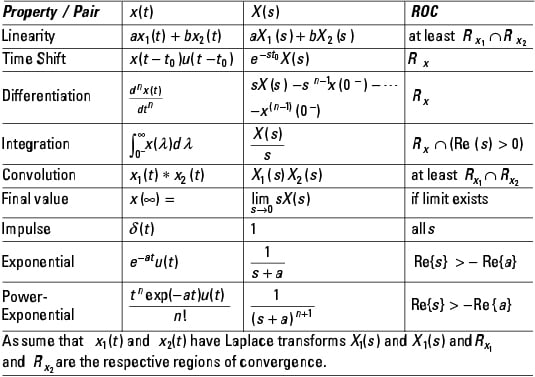## Letting the z-Transform help with signals and systems analysis

For discrete-time signals and systems, the z-transform (ZT) is the counterpart to the Laplace transform. With the ZT you can characterize signals and systems as well as solve linear constant coefficient difference equations. The two-sided ZT is defined as: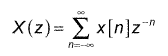The inverse ZT is typically found using partial fraction expansion and the use of ZT theorems and pairs. Here is a short table of ZT theorems and pairs.